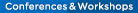# Event info

## Conference: "Statistics and Mathematical Modelling in Combination"Conference: "Statistics and Mathematical Modelling in Combination"

Conference: "Statistics and Mathematical Modelling in Combination"

16 - 18 November 2022

Contributed talks and invited lectures

Contributed talks may relate to but are not limited to the following topics:

1. Model identification, where statistical methods are used to deduce the coefficients of differential equations from a set of output data.
2. Inverse problems to determine transport coefficients in diffusion models from noisy data.
3. Evolution equations with uncertain model parameters.
4. Transport in random media.
5.Partial differential equations for pricing financial derivatives based on fluctuating stocks.
6. Stationary distributions of ergodic dynamical systems.
7. Path integrals to represent stochastic dynamical systems.
8. Boltzmann equation based on statistical dynamics.
9. Fractional-order differential equations to represent transport in fractal media.
10. Modelling real ecosystems.
11. Modelling complex economic systems.
12. Statistical change point identification for switching dynamical models.
13. Problems in the probabilistic interpretation of deterministic Schroedinger equation.

-Full registration AU 0
-Members of Aust. Math. Soc. and Members of AMSI member institutions AU
-Students and unemployed AU

Note: Members of overseas societies with reciprocal agreements can join Aust. Math. Soc. with free membership in the first year. Registrants will be served light lunches. registration coming soon.

Inquiries initially to: R.Chisholm@latrobe.edu.au

Flyer for Maths Forum（190 KB）

### News & Events